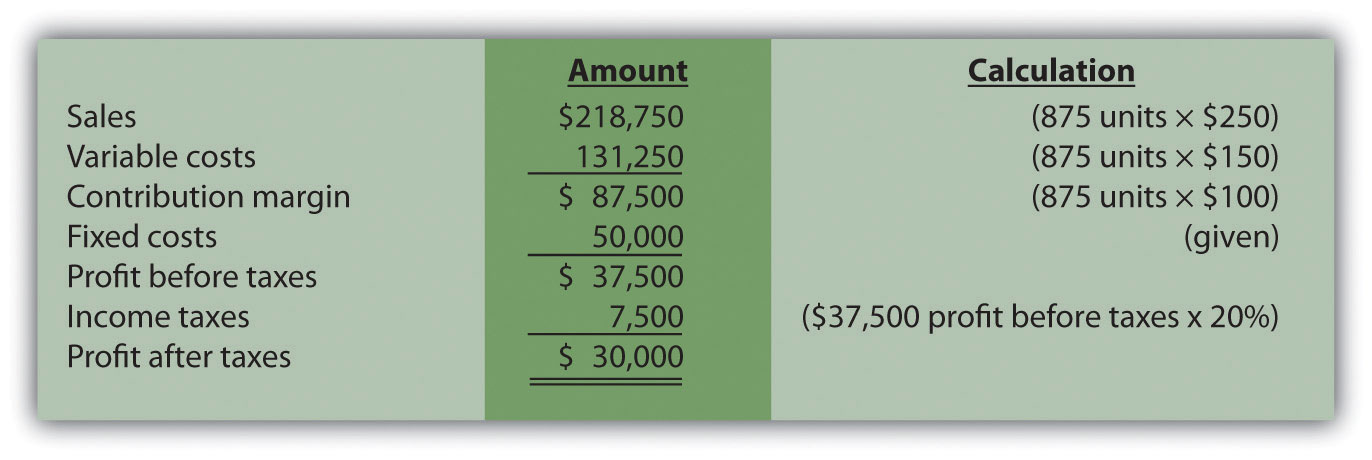## 6.6 Income Taxes and Cost-Volume-Profit Analysis

### Learning Objective

1. Understand the effect of income taxes on cost-volume-profit analysis.

Question: Some organizations, such as not-for-profit entities and governmental agencies, are not required to pay income taxes. However, most for-profit organizations must pay income taxes on their profits. How do we find the target profit in units or sales dollars for organizations that pay income taxes?

Step 1. Determine the desired target profit after taxes (i.e., after accounting for income taxes).

Step 2. Convert the desired target profit after taxes to the target profit before taxes.

Step 3. Use the target profit before taxes in the appropriate formula to calculate the target profit in units or sales dollars.

Using Snowboard Company as an example, the assumptions are as follows:

 Sales price per unit $250 Variable cost per unit 150 Fixed costs per month 50,000 Target profit 30,000 Assume also that the$30,000 target profit is the monthly profit desired after taxes and that Snowboard has a tax rate of 20 percent.

Step 1. Determine the desired target profit after taxes.

Snowboard’s management wants to know how many units must be sold to earn a profit of $30,000 after taxes. Target profit before taxes will be higher than$30,000, and we calculate it in the next step.

Step 2. Convert the desired target profit after taxes to the target profit before taxes.

The formula used to solve for target profit before taxes is as follows.

### Key Equation

Target profit before taxes = Target profit after taxes ÷ (1 − tax rate)

Using Snowboard Company’s data, the formula would read as follows:

Step 3. Use the target profit before taxes in the appropriate formula to calculate the target profit in units or sales dollars.

The formula used to solve for target profit in units is

For Snowboard Company, it would read as follows:

This answer is confirmed in the following contribution margin income statement.### Key Takeaway

• Companies that incur income taxes must follow three steps to find the break-even point or target profit.

Step 1. Determine the desired target profit after taxes.

Step 2. Convert the desired target profit after taxes to target profit before taxes using the following formula:

Target profit before taxes = Target profit after taxes ÷ (1 − tax rate)

Step 3. Use the target profit before taxes from step 2 in the appropriate target profit formula to calculate the target profit in units or in sales dollars.

### Review Problem 6.7

This review problem is based on the information for Snowboard Company. Assume Snowboard’s tax rate remains at 20 percent.

1. Use the three steps described in this section to determine how many units Snowboard Company must sell to earn a monthly profit of $50,000 after taxes. 2. Use the three steps to determine the sales dollars Snowboard needs to earn a monthly profit of$60,000 after taxes.

Solution to Review Problem 6.7

1. The three steps to determine how many units must be sold to earn a target profit after taxes are as follows:

Step 1. Determine the desired target profit after taxes.

Management wants a profit of $50,000 after taxes and needs to know how many units must be sold to earn this profit. Step 2. Convert the desired target profit after taxes to the target profit before taxes. The formula used to solve for target profit before taxes is Step 3. Use the target profit before taxes in the appropriate formula to calculate the target profit in units. The formula to solve for target profit in units is For Snowboard Company, it would read as follows: 2. The three steps to determine how many sales dollars are required to achieve a target profit after taxes are as follows: Step 1. Determine the desired target profit after taxes. Management wants a profit of$60,000 after taxes and needs to know the sales dollars required to earn this profit.

Step 2. Convert the desired target profit after taxes to target profit before taxes.

The formula used to solve for target profit before taxes is

Step 3. Use the target profit before taxes in the appropriate formula to calculate the target profit in sales dollars.

The formula used to solve for target profit in sales dollars is